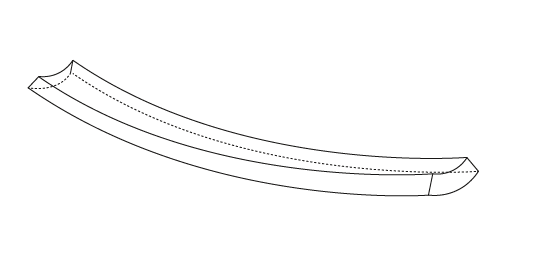# Precision modeling bend objectsI need some advice how to model an object like this. It has to be quite precise.

It has to be quite precise.
What does this mean ? Is it precise or not, and if not precise, how precise ? How is the curve defined, by an equation or is a reference image sufficient ?

If it doesn’t have to match the mathematical equation for the curve, add a background image showing the curve you want and the cross section shape, add two bezier curves to match each and use one as the bevel object of the other, convert to a mesh